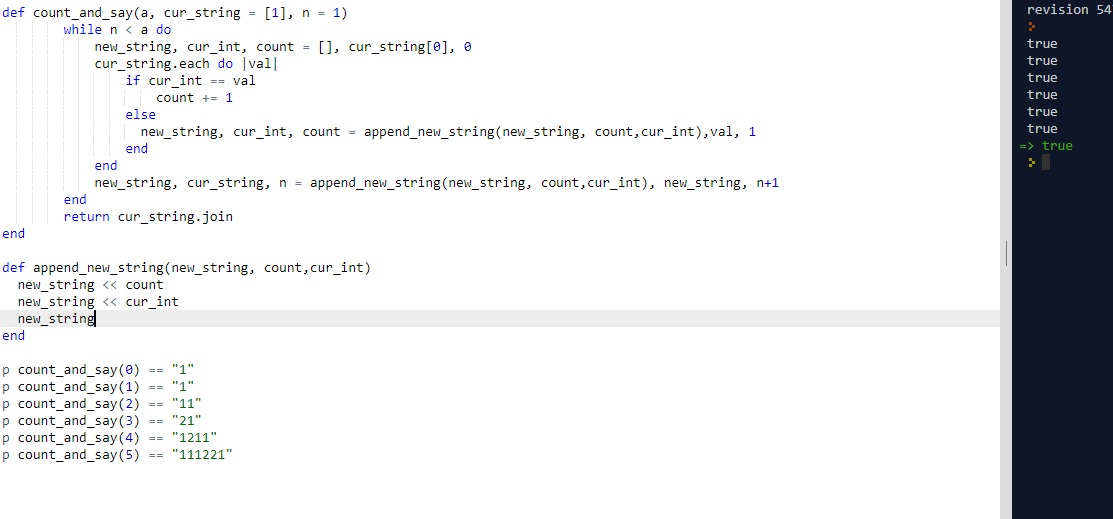# (*) CLEAN CODE: COUNT AND SAY

By: Saurav

2017-11-09 21:25:00 UTC

The count-and-say sequence is the sequence of integers beginning as follows:

1, 11, 21, 1211, 111221, ...
1 is read off as one 1 or 11.
11 is read off as two 1s or 21.

21 is read off as one 2, then one 1 or 1211.

Given an integer n, generate the nth sequence.

Note: The sequence of integers will be represented as a string.

Example:

if n = 2,
the sequence is 11.

InterviewBIt

Lets start with writing some tests first:

```p count_and_say(0) == "1"
p count_and_say(1) == "1"
p count_and_say(2) == "11"
p count_and_say(3) == "21"
p count_and_say(4) == "1211"
p count_and_say(5) == "111221"```

I started thinking with a recursive approach to solve this question.
Since, the question itself says one has to find the present number based on reading the last one:

For example:
 -> next would be 11 (One one, which reads what is previous)
[1, 11] -> next would be 21 (two one, which reads what is previous)
[1, 11, 21] -> next would be 1211 (One two and one one, which reads what is previous) and so on.

I thought of writing the solution with recursion then thought of simple iterative approach of storing the results in an array instead. Recursion uses stack, why not use an array instead to store all numbers from 1 to n

1st attempt (with help from geeks for geeks and Interviewbit)

```def count_and_say(a)
cur_string = 
n = 1
while n < a do
new_string = []
cur_int = cur_string
count = 0
cur_string.each do |val|
if cur_int == val
count += 1
else
new_string << count
new_string << cur_int
cur_int = val
count = 1
end
end
new_string << count
new_string << cur_int
cur_string = new_string
n += 1
end

cur_string.join
end```

2nd Attempt

```def count_and_say(a, cur_string = , n = 1)
while n < a do
new_string, cur_int, count = [], cur_string, 0
cur_string.each do |val|
if cur_int == val
count += 1
else
new_string, cur_int, count = append_new_string(new_string, count,cur_int),val, 1
end
end
new_string, cur_string, n = append_new_string(new_string, count,cur_int), new_string, n+1
end
return cur_string.join
end

def append_new_string(new_string, count,cur_int)
new_string << count
new_string << cur_int
new_string
end ```As we see, all our tests pass and the code looks clean as well.

I faced some problems with the recursive solution. I will update the recursive solution as well afterwards.

### Let me know what you think! twitter: sprakash24octlinkedin

Owned & Maintained by Saurav Prakash

If you like what you see, you can help me cover server costs or buy me a cup of coffee though donation :)